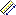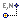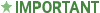# Measure - Intergraph Smart 3D - Help

## Intergraph Smart 3D Common

PPMProduct
Intergraph Smart 3D
PPMCategory_custom
Help
Version_S3D
10.1(2014R1)Measures distances and angles between objects in the model, including edges, faces, and key points.

With the Tools > Measure command, you can:

• Measure the actual 3D linear distance between two points.

• Measure the delta E- (X), N- (Y), and EL- (Z) distance using the last active coordinate system defined in the PinPointor Measure commands.

• Measure distance along an object, like the Point Along command, or the entire length of an object.

• Measure minimum distance between two objects, using the outside surface and not just the axis.

• Measure the minimum distance between two objects as projected to a selected plane.

• Measure and display hole radius and diameter as well as measure and display fillet radius.

• Measure the actual angle defined by three points.

• Measure angle between lines, using cylinder axes or nozzle axes as reference lines.

• Find SmartSketch points when the software prompts you to locate a start or end point to measure.

• Copy measurement values from the ribbon. The Measure command also sums repeated measurements and displays the cumulative results on the ribbon.

When you move the pointer over a key point, the distance between the current location of the pointer and the last point that you clicked displays next to the pointer in text and on the ribbon along with the delta values. The delta values are the distances, as measured along the E- (X), N- (Y), and EL- (Z) axes.

You can change the displayed units of measure for distance or angle by using the Tools > Options command.You can use the Measure command to set the active coordinate system, which is a temporary coordinate system with a new origin and axis directions different from those of the global coordinate system. The active coordinate system affects certain calculations, such as weight and CG.

Measure Ribbon

What do you want to do?